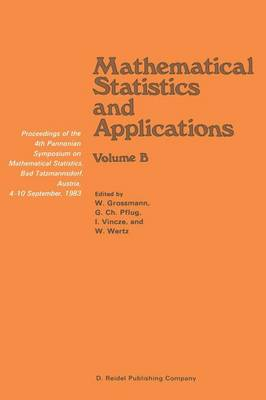•# Mathematical Statistics and Applications: Proceedings of the 4th Pannonian Symposium on Mathematical Statistics, Bad Tatzmannsdorf, Austria, 4-10 September, 1983 Volume B (Paperback)

(editor), (editor), (editor), (editor)
£109.99
Paperback 328 Pages / Published: 02/10/2011
• We can order this from the publisher

UK delivery within 3-4 weeks

The Fourth Pannonian Symposium on Mathematical Statistics was held in Bad Tatzmannsdorf, Austria, 4-10 September, 1983. The first two Symposia were held there in 1979 and 1981; whereas the Third Symposium was staged in Visegrad, Hungary in 1982. The proceedings volumes of these conferences, published by Springer, D. Reidel, and D. Reidel & Akademiai Kiad6, respec tively give information about the objectives of the Pannonian Symposia and the topics covered. About 130 participants from 17 countries took part in this Fourth Symposium, and 92 lectures were presented. This volume contains 21 reviewed contributions which cover various aspects of the application of mathematical statistics. A second group of papers dealing with problems of probability theory and decision theory is published in a separate volume entitled "Probability and Statistidal Decision Theory". Roughly speaking, the papers can be grouped into three main categories. The first group is the application of probability theory. A special type of application is shown in the invited paper of P. Erdos, namely probabilistic methods in number theory. Further models of applied probability covered by the papers are game theory, urn models, best choice models and random graphs. The second group could be best characterized by the term mathematical statistics for models of real data. Such models are linear models, regression, discrimination, time series, analysis of censored data, goodness of fit approxima tion of processes. The papers show the increasing importance of VII new theoretical results (i. e.

Publisher: Springer
ISBN: 9789401089012
Number of pages: 328
Weight: 510 g
Dimensions: 235 x 155 x 17 mm
Edition: Softcover reprint of the original 1st ed. 198